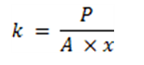# Calculating Spring Constant for Attachment

## Designing Heatsinks and Thermal Solutions for Xilinx Devices (XAPP1377)

Document ID
XAPP1377
Release Date
2022-06-06
Revision
1.0 English

To properly size the spring constants for applying the 20 to 50 PSI of pressure to one of our lidded or lidless devices, we first need to calculate how much force is needed to determine which springs can provide that force. The equation for force is relatively simple: force = pressure × area. The following steps show you how to find the force in pounds for our example.

1. Convert the units from mm into pounds by dividing the calculated mm2 surface area calculated earlier by 645.16 to covert to inches2.
2. Multiply that area by the desired pressure (generally in the mid-range of the device pressure specification) to find the target force.
3. Divide that number by the number of attachment screws to get the force per screw. This force can be used to determine the spring constant needed based on the desired compression of the spring.
Note: Take special care to ensure all units are converted to compatible formats to ensure proper calculation.

After a spring is selected, we suggest that you back-calculate the pressure the springs will apply on the device to ensure the minimum or maximum pressure specification is not violated. The simple equation to calculate the necessary spring constant (k) is as follows.

Figure 1. Necessary Spring Constant (k)Where:
• k is the spring constant we are solving for in pound-force
• P is target pressure in PSI
• A is calculated surface area to the device in inches2
• x is the spring compression in inches